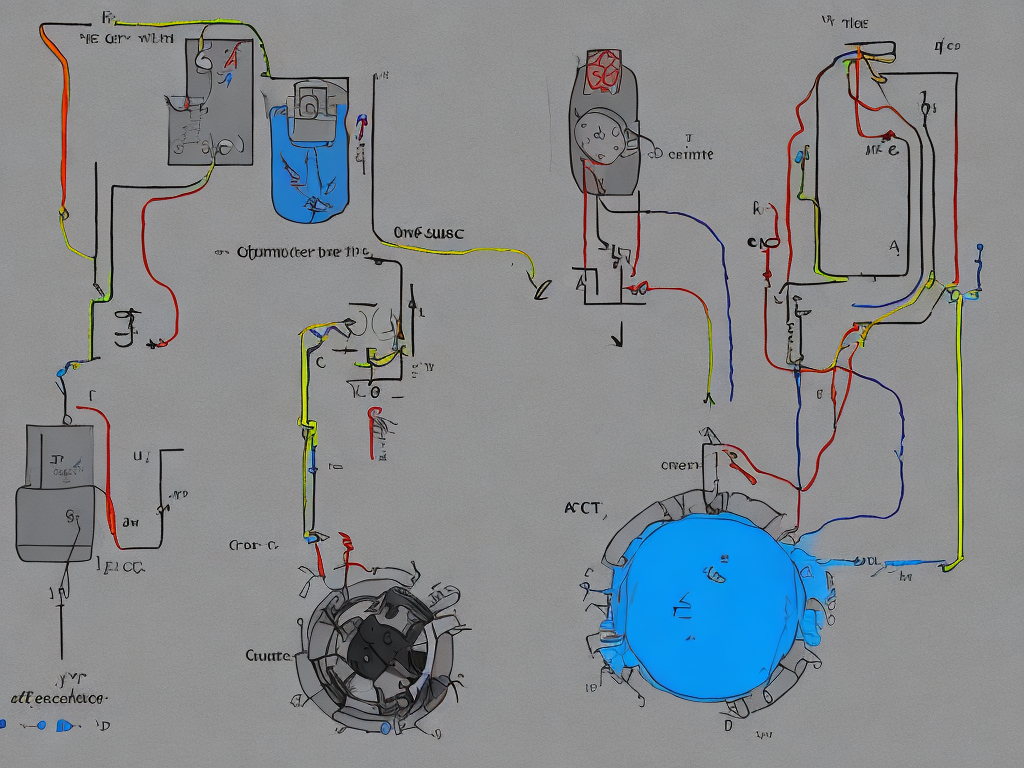# Difference Between Ac And Dc Current Class 10

tl;dr
The main difference between AC and DC current is the direction of the flow of electric charge, as well as their frequency, magnitude, efficiency, uses, safety concerns, and voltage drop.Electricity is the flow of electric charge in a conductor. There are two types of electric currents: direct current (DC) and alternating current (AC). In this article, we will discuss the differences between DC and AC current in depth.

Direct Current (DC)

Direct current (DC) is the movement of electric charges in a single direction. The flow of current is in one direction from positive to negative. DC current is used mostly in batteries, fuel cells, and electronic devices like cell phones, mp3 players, laptops, etc that use direct current to operate.

A direct current voltage source produces a steady voltage that remains constant over time. The voltage value never changes in a complete circuit in DC. The frequency of DC is zero, the polarity of voltage remains the same and there is no sinusoidal wave produced by DC.

The magnitude of DC can be positive, negative, or zero. The DC magnitude is measured in volts (V) or millivolts (mV). This type of current is also used in some electrical devices that require a low power supply to operate.

Alternating Current (AC)

Alternating Current (AC) is the flow of electric charges in a circuit that periodically reverses its direction. The voltage changes polarity over time, and hence the direction of the current also changes after every half-cycle.

AC generators are used to produce AC. The voltage is generated by multiple coils of wire, which are rotated by a turbine. The voltage produced by each coil is added together, resulting in an AC voltage that is stable and can be transmitted over long distances.

The frequency of AC is the rate at which the voltage alternates in one direction to the other. The most common frequency for AC in the US is 60 Hz (Hertz), where Hertz is the unit of frequency (number of cycles per second). In Europe, the frequency is 50 Hz, and it varies in other countries.

The magnitude of AC current and voltage is measured in RMS, which is the root mean square value of the AC waveform. The RMS value of AC is the equivalent DC value that produces the same heating effect in a resistor that the AC produces in its full alternating cycle.

The voltage waveform of AC resembles a sinusoidal wave or sine wave. This type of waveform is used because it is the simplest waveform to produce and also because most electrical devices can operate on AC.

Difference Between AC and DC Current

The main difference between AC and DC current lies in the direction of the flow of electric charge. In DC, the voltage source produces a constant voltage that does not change over time, while in AC, the voltage changes polarity over time, reversing the direction of the current flow.

The other differences between AC and DC current are:

1. Frequency: AC has a frequency, while DC has zero frequency. The frequency of AC is measured in Hertz (Hz), while DC has no frequency.

2. Magnitude: The magnitude of AC current and voltage is measured in RMS value, while the magnitude of DC is measured in volts or millivolts.

3. Energy Efficiency: AC is more efficient than DC in terms of energy transmission over long distances. AC voltage can easily be transformed to higher or lower voltages, while DC requires a much more expensive process to transform the voltage.

4. Uses: DC is used in electronic devices, batteries, and fuel cells, while AC is used for power transmission in homes and businesses.

5. Safety Concerns: DC is generally considered safer for human exposure than AC. AC is more dangerous because its frequency is within the range of human nerves, which means that it can easily stimulate the human nervous system and cause fatal accidents.

6. Voltage Drop: AC voltage can be transmitted over long distances with relatively low voltage drop, while DC voltage drops rapidly over long distances.

Conclusion

In conclusion, the main differences between AC and DC are the direction of the flow of electric charge, frequency, magnitude, efficiency, uses, safety concerns, and voltage drop. Understanding the differences between these two types of current is important when dealing with electrical devices and systems. It is important to know which type of current to use in different applications and to take necessary safety precautions when working with electricity.